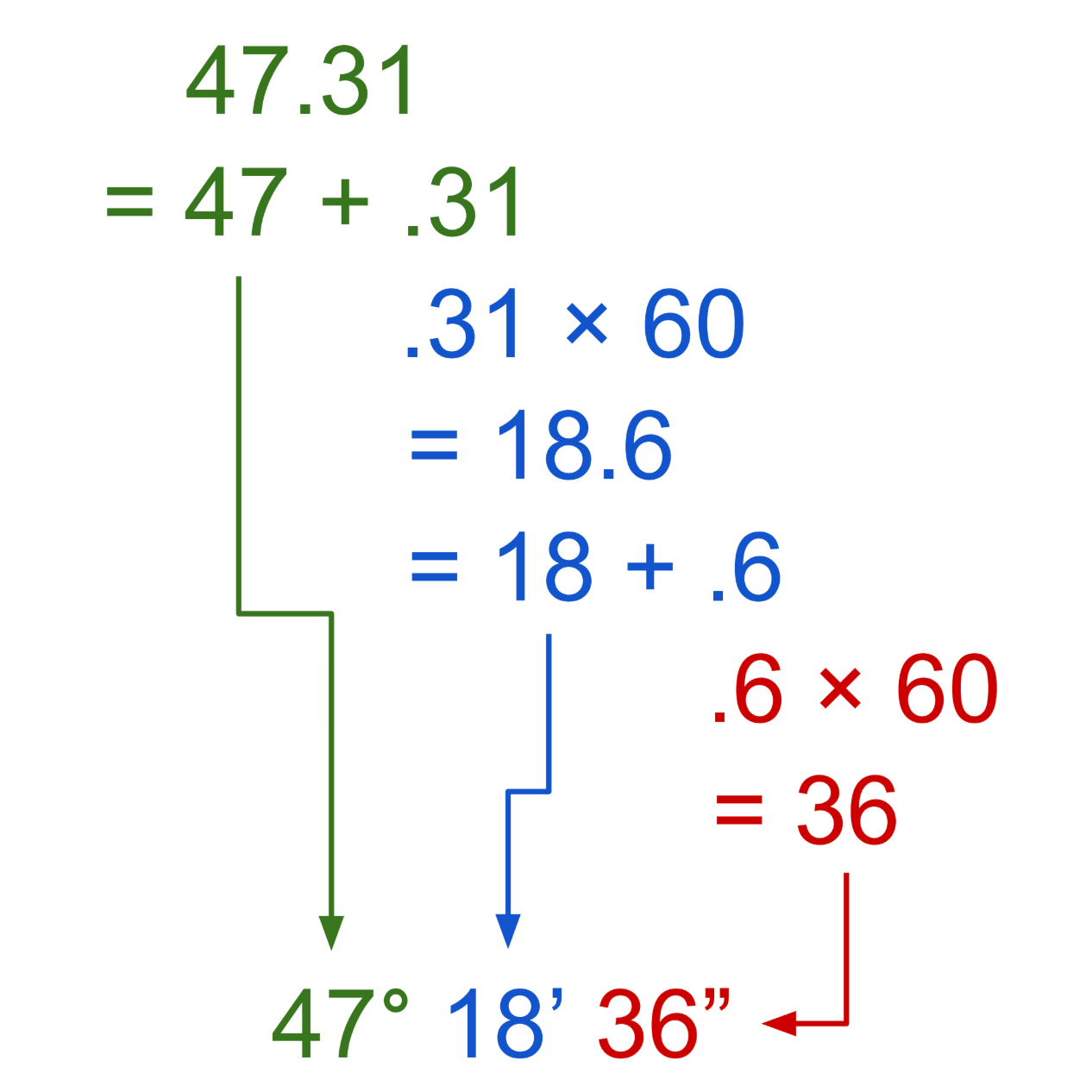# Degrees Decimal to Degrees, Minutes, and Seconds Conversion Calculator

Enter degrees in decimal to convert to minutes and seconds. See the formulas below to see how it’s done.

Angle:
°

## Degrees, Minutes, & Seconds:

47° 18′ 36″

Try our calculator to convert degrees, minutes, and seconds to decimal.

## How to Convert Degrees as a Decimal to Degrees, Minutes, & Seconds

In addition to decimal, degrees can also be expressed with minutes and seconds.
Typically, minutes (′) are used to express an angle that is smaller than one degree. Seconds (″) are used to express an angle that is smaller than one minute.

To convert degrees in decimal form to degrees with minutes and seconds, follow a few simple steps.

Since minutes and seconds are used to express the measurement of an angle that is smaller than one degree, start by setting the number to the left of the decimal point as the degrees.

The decimal point, or remainder of the angle that is smaller than one degree can be converted to minutes and seconds. Multiply this decimal value by 60 to find the number of minutes.

minutes = remaining decimal × 60

This resulting number is the minutes and seconds. The whole number to the left of the decimal point is the number of minutes. The remainder is the number of partial minutes, which need to be converted to seconds.

Multiply the remaining decimal value by 60 again to find the number of seconds.

seconds = remaining decimal × 60

Finally, express the whole degrees, minutes, and seconds with their respective symbols

deg° min′ sec″

For example, convert 47.31° to degrees, minutes, & seconds.

Find the whole degrees:
47.31° = 47° + .31°
degrees = 47°

Find minutes:
minutes = .31 × 60 = 18.6′
minutes = 18′ + .6′
minutes = 18′

Find seconds:
seconds = .6′ × 60
seconds = 36″

Thus, 47.31° is equal to 47° 18′ 36″.Here’s a fun fact: there are 360 degrees in a circle, and each degree is composed of 60 minutes or 3,600 seconds. That means that there are 21,600 minutes around a circle and 1,296,000 seconds around a circle.